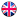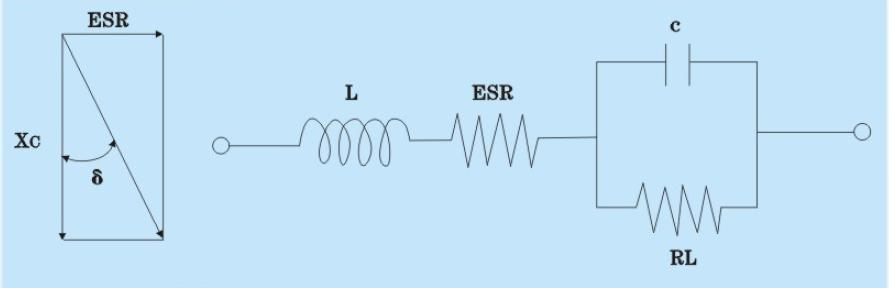EnglishHot keywords :

#### Basic Descriptiion of SMD/Chip Tantalum Capacitors

2016-05-12

1. Capacitance

Cn said capacitance that unit for uF, appy 100Hz or 120Hz series equivaletnt circuit to do measurements in order to avoid the effects of power frequency. Standard testing voltage U_=U_=2.20 -1.0V (effective value) or lower, temperature is 25,  and 15~35range is allowed

2. Tolerance

Tolerance indicate the value difference of capacitance that allowed

3. Loss Angle Tangent Value tgδ
Considering resistance is exist in capacitors, the electric device response on capacitors Xc=1/2πfc and Equivalent Series Resistance ESR to indicate wastage as tgδ=ESR/Xc in series connected circuit.
loss angle tangent value is measured under 0.5VAC 120Hz and write in percentage.4. Rated Voltage

Indicate the max DC voltage can be applied continuously on capacitors.  Use VR / VR to say it with unit as volts (V).

5. Leakage Current
It required to connect 1 resitance to measure leakage and apply rated voltage 5mins. Standard leakage current should not above the result of capacitance multiply rated voltage and a constant.

6. Equivalent Series Resistance
Equivalent series resitance is measured in loop circuit of capacitors with freqency 100KHz.

7. Using Temperature

Using temperature range is-55~125and max using temperature is +85under rated voltage. When it is above 85category voltages which are indicated in datasheet are allowed to apply. Category voltage is about 0.65 tims of rated voltage.